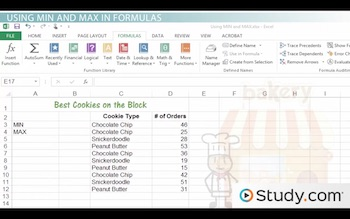# Using the MIN and MAX Functions in Excel

An error occurred trying to load this video.

Try refreshing the page, or contact customer support.

Coming up next: Using the COUNT Function in Excel

### You're on a roll. Keep up the good work!

Replay
Your next lesson will play in 10 seconds
• 0:02 MIN & MAX Functions
• 0:40 Using MIN & MAX in Formulas
• 1:42 Using Single Cells…
• 2:54 Combining with the IF Function
• 6:05 Lesson Summary
Save Save

Want to watch this again later?

Timeline
Autoplay
Autoplay
Speed Speed Audio mode

#### Recommended Lessons and Courses for You

Lesson Transcript
Instructor: Karen Sorensen

Karen has a Bachelors in Communications. She has 25 years of experience in Information Systems, Adult Learning and Virtual Training.

Looking in a cell range of numbers to find the smallest number, or the largest, can be time consuming and inefficient. This lesson will introduce you to the MIN and MAX functions in Excel and demonstrate how to use them in formulas.

## MIN and MAX Functions

The MIN and MAX functions are just what the names imply. MIN will find the lowest number in a range, while MAX finds the largest number in a range. One advantage to these functions is that they can be combined with the IF function. Combining functions means writing a formula that is a bit more complex, but is nevertheless handy in some worksheets.

In this lesson, you will learn how to use the MIN and MAX functions. We will take a look at using them in formulas. And, we will also look at examples where the MIN and MAX functions are used in combination with the IF function.

## Using MIN and MAX in Formulas

Let's begin. Imagine you own a cookie-making business, Best Cookies on the Block. You created a worksheet, and over the last eight weeks you tracked the total orders for each cookie. Now, you need a couple formulas. You want to see the largest and the smallest orders.Looking at the example worksheet, you have entered the cookie names in column C and the number of orders is in column D. In cells B3 and B4, you would like to insert the formulas using MIN and MAX. Let's start with the MIN formula in B3. This would be =MIN(D3:D12). The result will be the number that is the smallest. Then, in B4, to determine the largest order, we can enter a similar formula using the MAX function: =MAX(D3:D12). Perfect. Let's keep going.

## Using Single Cells References and Numbers

In the previous topic, we looked at example formulas using a cell range, such as D3:D12. However, you can also use a single cell reference and/or actual numbers in your formulas. You can also add other operators and calculations to the formula. Let's take a look at another example.

Assume that you already know your top three cookies: chocolate chip, peanut butter and the gingersnap. The order totals are in D3, D6 and D11, respectively. You want the formula to look in only those three cells, rather than the entire range. Let's use the MAX function and find the largest order among the top three. So, we would enter =MAX(D3,D6,D11).

Notice that you can enter individual cell references, rather than an entire range. You could also write the formula with numbers and cell references, such as =MAX(D3,D6,51). Okay, moving on to something a bit more complex.

## Combining with the IF Function

I want to take the MIN and MAX functions one step further and use them in combination with the IF function. These type of formulas are more complex, but I will break it down to simplify the process. Going back to our worksheet of cookie orders, let's assume that you want to see the MAX for one particular cookie type: chocolate chip. For instance, the largest order for chocolate chip cookies.

Now, you can definitely type in each individual cell reference for the chocolate chip cookies, such as =MAX(D3,D4,D7,D10). But imagine the worksheet is filled with data, with a long list of orders for chocolate chip cookies. Identifying each cell reference in order to write the formula would be time consuming. Especially when we can write a formula that will look for and find just the chocolate chip orders, and then tell you what total is the largest among just those orders.

To unlock this lesson you must be a Study.com Member.

### Register to view this lesson

Are you a student or a teacher?

#### See for yourself why 30 million people use Study.com

##### Become a Study.com member and start learning now.
Back
What teachers are saying about Study.com

### Earning College Credit

Did you know… We have over 200 college courses that prepare you to earn credit by exam that is accepted by over 1,500 colleges and universities. You can test out of the first two years of college and save thousands off your degree. Anyone can earn credit-by-exam regardless of age or education level.